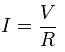# Electricity Basics

Current and Voltage

Electricity is a flow of electrons.

If we can measure the electricity, we must therefore be able to say how many electrons are in the flow. We measure amount of electricity, as a charge in coulombs.

Electron flow rate is similar to volume of water which flow through a water pipe during a defined period of time. Electron flow rate is called current (denoted as ‘I’). Electric current is measured in amperes (short form is ‘amps’, or ‘A’), where one amp is defined as a quantity of one coulomb passing a point in one second.

With electricity the flow pressure is defined by the difference in numbers of electrons between two points called as potential difference or Voltage (denoted by ‘V’ and units are volts). Voltage is simply a way of expressing electrical pushing power.

If you know the amps and volts involved, you can determine the amount of electricity consumed, which we measure in watts

Ohm’s Law

There is a relationship between pushing power in volts and the rate of electron flow in amps. After all, the higher the voltage, the more pushing power the electrons have behind them so faster they should flow. This relationship is known as Ohm’s law. It may be summarized as

“The current through a conductor between two points is directly proportional to the potential difference across the two points, and inversely proportional to the resistance between them.”

The mathematical equation isWhere I is the current through the conductor in units of amperes, V is the potential difference measured across the conductor in units of volts, and R is the resistance of the conductor in units of ohms. More specifically, Ohm’s law states that the R in this relation is constant, and the constant is commonly called the substance’s resistance (as, it is a measure of the amount the substance resists the flow of current through it) and is given the unit: Ω (pronounced ohm).

Power

AC and DC

In direct current (DC) the flow of electric charge is only in one direction i.e. possessing a voltage with constant polarity over time. It is produced by batteries, thermocouples and solar cells.

In alternating current (AC) the movement of electric charge periodically reverses direction so, voltage alternates in polarity i.e. reversing positive and negative over time.

AC is the form in which electric power is delivered. The usual waveform of an AC power circuit is a sine wave.

Phase, neutral and ground

Phase wire is an electrically charged, conductive wire that provides power to the load. The phase wire is usually covered with red insulation.

Neutral wire is the wire that conducts the power back to the source and completes the circuit and is the return path for current supplied by the phase wire. The neutral wire is usually covered with white insulation.

The ground wire is the extra wire in an electrical system that provides a low-resistance path to ground for stray current. It is not supposed to carry any current at all until a fault of some kind occurs and covered with green insulation.

Grounding

Grounding of equipment refers to connecting the non-current-carrying metal parts of the wiring system or equipment to ground so, the resistance of the path through the grounding conductor will usually be much less than the resistance through the stray path, and not much current will flow through the stray path. Sufficient current will usually flow through the grounded path to blow the circuit fuse or trip the circuit breaker and thus open the circuit. On the other hand, if the equipment is not grounded, sufficient current will flow through the stray path to be a shock hazard.

## Apply for IT Support Certification

https://www.vskills.in/certification/certified-it-support-professional

#### Back to Tutorials